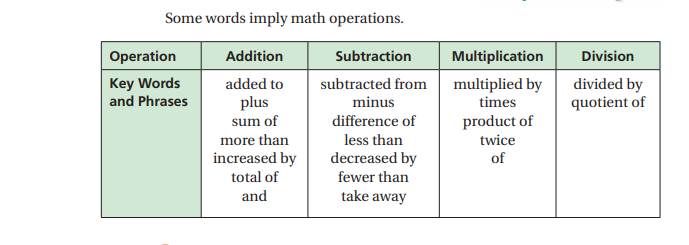## Current Math Topic

January - February: Your child is learning about Equations. An equation is a math sentence that contains: variables, constants, and coefficients and an equal sign.

This is the beginning of Algebra for your student! Kids are working with hands on equations, which are manipulatives that help them "balance" a written equation. Please ask your child to explain how hands on equations work!

December: Your child is learning about math properties. Properties to memorize are:

Commutative Property of Addition and Multiplication
Associative Property
Addition Property of Zero
Multiplication Property of Zero and One

We are currently working on how to write algebraic expressions. An algebraic expression is a math sentence. Students must be able to identify words that mean add, subtract, multiply and divide. Kids can use the chart below to help them write the expressions!New vocabulary words to know!

Algebraic Expression Terms
Variable Like-terms
Constant Operation (Operator)
Coefficient Evaluate